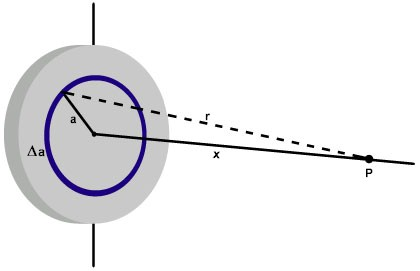# The flight to the center of the discA particle $P$ having a charge of $-1\ \mu\text{C}$ and mass 2 g is held at a distance of $x=1\text{ m}$ from the center of a disc, as shown in the figure above. The disc has a radius of 2 m and surface charge density $6.84\times 10^{-4} \text{ C/m}^2$.

The particle is released, and flies toward the disc. Find the velocity (in m/s) of the particle when it has covered half the distance.

×